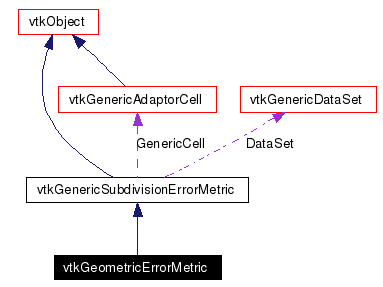# vtkGeometricErrorMetric Class Reference

`#include <vtkGeometricErrorMetric.h>`

Inheritance diagram for vtkGeometricErrorMetric:[legend]
Collaboration diagram for vtkGeometricErrorMetric:[legend]
List of all members.

## Detailed Description

Objects that compute geometry-based error during cell tessellation.

It is a concrete error metric, based on a geometric criterium: the variation of the edge from a straight line.

See also:
vtkGenericCellTessellator vtkGenericSubdivisionErrorMetric
Tests:
vtkGeometricErrorMetric (Tests)

Definition at line 37 of file vtkGeometricErrorMetric.h.
typedef vtkGenericSubdivisionErrorMetric Superclass
virtual const char * GetClassName ()
virtual int IsA (const char *type)
void PrintSelf (ostream &os, vtkIndent indent)
static vtkGeometricErrorMetricNew ()
static int IsTypeOf (const char *type)
static vtkGeometricErrorMetricSafeDownCast (vtkObject *o)

## Public Member Functions

int GetRelative ()
virtual double GetAbsoluteGeometricTolerance ()
void SetAbsoluteGeometricTolerance (double value)
void SetRelativeGeometricTolerance (double value, vtkGenericDataSet *ds)
int RequiresEdgeSubdivision (double *leftPoint, double *midPoint, double *rightPoint, double alpha)
double GetError (double *leftPoint, double *midPoint, double *rightPoint, double alpha)

## Protected Member Functions

vtkGeometricErrorMetric ()
virtual ~vtkGeometricErrorMetric ()
double Distance2LinePoint (double x, double y, double z)

## Protected Attributes

double AbsoluteGeometricTolerance
double SmallestSize
int Relative

## Member Typedef Documentation

 Standard VTK type and error macros. Reimplemented from vtkGenericSubdivisionErrorMetric. Definition at line 46 of file vtkGeometricErrorMetric.h.

## Constructor & Destructor Documentation

 vtkGeometricErrorMetric::vtkGeometricErrorMetric ( ) ` [protected]`

 virtual vtkGeometricErrorMetric::~vtkGeometricErrorMetric ( ) ` [protected, virtual]`

## Member Function Documentation

 static vtkGeometricErrorMetric* vtkGeometricErrorMetric::New ( ) ` [static]`
 Construct the error metric with a default squared absolute geometric accuracy equal to 1. Reimplemented from vtkObject.

 virtual const char* vtkGeometricErrorMetric::GetClassName ( ) ` [virtual]`
 Standard VTK type and error macros. Reimplemented from vtkGenericSubdivisionErrorMetric.

 static int vtkGeometricErrorMetric::IsTypeOf ( const char * type ) ` [static]`
 Standard VTK type and error macros. Reimplemented from vtkGenericSubdivisionErrorMetric.

 virtual int vtkGeometricErrorMetric::IsA ( const char * type ) ` [virtual]`
 Standard VTK type and error macros. Reimplemented from vtkGenericSubdivisionErrorMetric.

 static vtkGeometricErrorMetric* vtkGeometricErrorMetric::SafeDownCast ( vtkObject * o ) ` [static]`
 Standard VTK type and error macros. Reimplemented from vtkGenericSubdivisionErrorMetric.

 void vtkGeometricErrorMetric::PrintSelf ( ostream & os, vtkIndent indent ) ` [virtual]`
 Standard VTK type and error macros. Reimplemented from vtkGenericSubdivisionErrorMetric.

 virtual double vtkGeometricErrorMetric::GetAbsoluteGeometricTolerance ( ) ` [virtual]`
 Return the squared absolute geometric accuracy. See SetAbsoluteGeometricTolerance() for details.Postcondition:positive_result: result>0

 void vtkGeometricErrorMetric::SetAbsoluteGeometricTolerance ( double value )
 Set the geometric accuracy with a squared absolute value. This is the geometric object-based accuracy. Subdivision will be required if the square distance between the real point and the straight line passing through the vertices of the edge is greater than `value'. For instance 0.01 will give better result than 0.1.Precondition:positive_value: value>0

 void vtkGeometricErrorMetric::SetRelativeGeometricTolerance ( double value, vtkGenericDataSet * ds )
 Set the geometric accuracy with a value relative to the length of the bounding box of the dataset. Internally compute the absolute tolerance. For instance 0.01 will give better result than 0.1.Precondition:valid_range_value: value>0 && value<1 ds_exists: ds!=0

 int vtkGeometricErrorMetric::RequiresEdgeSubdivision ( double * leftPoint, double * midPoint, double * rightPoint, double alpha ) ` [virtual]`
 Does the edge need to be subdivided according to the distance between the line passing through its endpoints and the mid point? The edge is defined by its `leftPoint' and its `rightPoint'. `leftPoint', `midPoint' and `rightPoint' have to be initialized before calling RequiresEdgeSubdivision(). Their format is global coordinates, parametric coordinates and point centered attributes: xyx rst abc de... `alpha' is the normalized abscissa of the midpoint along the edge. (close to 0 means close to the left point, close to 1 means close to the right point)Precondition:leftPoint_exists: leftPoint!=0 midPoint_exists: midPoint!=0 rightPoint_exists: rightPoint!=0 clamped_alpha: alpha>0 && alpha<1 valid_size: sizeof(leftPoint)=sizeof(midPoint)=sizeof(rightPoint) =GetAttributeCollection()->GetNumberOfPointCenteredComponents()+6 Implements vtkGenericSubdivisionErrorMetric.

 double vtkGeometricErrorMetric::GetError ( double * leftPoint, double * midPoint, double * rightPoint, double alpha ) ` [virtual]`
 Return the error at the mid-point. It will return an error relative to the bounding box size if GetRelative() is true, a square absolute error otherwise. See RequiresEdgeSubdivision() for a description of the arguments.Precondition:leftPoint_exists: leftPoint!=0 midPoint_exists: midPoint!=0 rightPoint_exists: rightPoint!=0 clamped_alpha: alpha>0 && alpha<1 valid_size: sizeof(leftPoint)=sizeof(midPoint)=sizeof(rightPoint) =GetAttributeCollection()->GetNumberOfPointCenteredComponents()+6 Postcondition:positive_result: result>=0 Implements vtkGenericSubdivisionErrorMetric.

 int vtkGeometricErrorMetric::GetRelative ( )
 Return the type of output of GetError()

 double vtkGeometricErrorMetric::Distance2LinePoint ( double x, double y, double z ) ` [protected]`
 Square distance between a straight line (defined by points x and y) and a point z. Property: if x and y are equal, the line is a point and the result is the square distance between points x and z.

## Member Data Documentation

 double vtkGeometricErrorMetric::AbsoluteGeometricTolerance` [protected]`
 Definition at line 122 of file vtkGeometricErrorMetric.h.

 double vtkGeometricErrorMetric::SmallestSize` [protected]`
 Definition at line 123 of file vtkGeometricErrorMetric.h.

 int vtkGeometricErrorMetric::Relative` [protected]`
 Definition at line 124 of file vtkGeometricErrorMetric.h.

The documentation for this class was generated from the following file:

Generated on Mon Jan 21 23:46:20 2008 for VTK by1.4.3-20050530